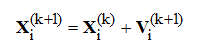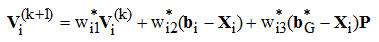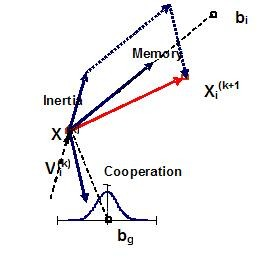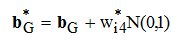##### Personal tools
You are here: Home The mutation scheme

# The mutation scheme

## EPSO recombination operator - the mutation scheme

Given a population with a set of particles, the general scheme of EPSO is the following:

• REPLICATION - each particle is replicated r times
• MUTATION - each particle has its strategic parameters mutated
• REPRODUCTION - each mutated particle generates an offspring through recombination, according to the particle movement rule, described below
• EVALUATION - each offspring has its fitness evaluated
• SELECTION - by stochastic tournament or other selection procedure, the best particles survive to form a new generation, composed of a selected descendant from every individual in the previous generation

Mutation of a parameter w into w* is ruled by multiplicative Lognormal random numbers such as in wi*=wi[logN(0,1)]τ , multiplicative Gaussian numbers such as in wi*=wi(1+σN(0,1))   or by additive Gaussian distributed random numbers such as in wi*=wi+σN(0,1) - not reccomended.The learning parameter (τ or σ) must be fixed externally.

The recombination rule for EPSO becomes (see Figure 1):where:

Xi(k) - location of particle i at generation k

Vi(k)=Xi(k)-Xi(k-1) - is the velocity of particle i at generation k

bi - best point found by particle i in its past life up to the current generation

bG - best overall point found by the swarm of particles in their past life up to the current generation

wi1 - weight conditioning the inertia term

wi2 - weight conditioning the memory term

wi3 - weight conditioning the cooperation or information exchange term

P - communication factor matrix (discussed below)Figure 1 – Illustration of the EPSO movement rule. Notice that the vector associated with the cooperation factor does not point exactly to the global optimum bg but to a mutated location.

The symbol * indicates that the parameter will undergo mutation. In the most effective EPSO variant, not only the weights affecting the components of movement are mutated but also the global best is randomly disturbed to givewhere wi4 is the forth strategic parameter (target weight) associated with particle i. It controls the “size” of the neighborhood of  bG where it is more likely to find the real global best solution.

The communication factor P is a diagonal matrix whose diagonal elements are 0 or 1 depending on a threshold communication probability. each time an element is needed, this threshold is compared against a random number Rnd() - uniform distribution - and the value of 0 or 1 is selected depending on Rnd() being smaller or greater than the fixed threshold.

Selection is modeled from the Stochastic Tornament concept: among the ofspring of each particle, one compares the best one with another particle randomly sampled, and the best is selected with probability (1 – luck), where the luck parameter is defined in [0,1] but is usually small. If luck = 0 we have elitist selection.

##### Document Actions
Contact

INESC TEC
Campus da FEUP
Rua Dr. Roberto Frias
4200 - 465 Porto
Portugal

Tel. +351 22 209 4000
Fax +351 22 209 4050